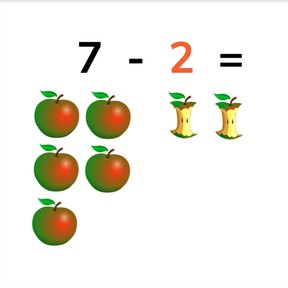Subtraction to 10 with 1 or 2

# Subtraction to 10 with 1 or 2

No account needed.8,000 schools use Gynzy92,000 teachers use Gynzy1,600,000 students use Gynzy

## General

Students learn subtracting with numbers to 10 by taking away 1 or 2.

1.OA.C.5
1.OA.C.6
1.OA.A

## Relevance

It is important to be able to do this, so you can determine how many you have left when you take 1 or 2 away.

## Introduction

The interactive whiteboard shows various animals next to a number. Drag the same number of animals into the box to the right of the number.

## Development

Using the images on the interactive whiteboard explain that you can subtract by counting back one from a given number. Count the total amount of fish in the pond, drag one fish from the pond to the bucket and then count how many fish are left. Next show how you can subtract with showing a number instead of erasing or moving one of the objects. You still take 1 away. Repeat this explanation first with images and then with the number for subtracting 2. To check that students can subtract with 1 or 2, do the given interactive whiteboard exercises together. Finally practice subtraction with 1 or 2 when the subtraction problems are entirely abstract, so only numbers showing. Emphasize to students that this process is exactly the same, you count back 1 or 2 from the first number (minuend).

To check that students understand subtraction to 10 with 1 or 2, you can ask the following questions:
- What does the minus sign (-) mean?
- How do you solve 8 - 1?
- How do you solve 5 - 2?

## Guided practice

Students first practice subtracting with 1 or 2 with support of visual images they can count. Next they practice subtraction shown in the abstract taking 1 away, followed by taking 2 away.

## Closing

Discuss with students that it is important to be able to subtract 1 or 2 from numbers to 10 so that you can determine how much you have left after taking 1 or 2 away. The interactive whiteboard shows 8 burning candles. Students are asked to determine how many candles are still burning if you blow one candle out. Ask students to come up with the subtraction problem that represents the image shown. Next they are shown six candies, and told that two are eaten. Students must determine how many candies are left as well as the subtraction problem that represents the image.

## Teaching tips

Students who have difficulty with this learning goal can be given blocks or other manipulatives to help visualize the subtraction process. They can then manually remove one or two blocks and count how many are left.

## Instruction materials

Optional: blocks or other manipulatives

### The online teaching platform for interactive whiteboards and displays in schools

• Save time building lessons

• Manage the classroom more efficiently

• Increase student engagement